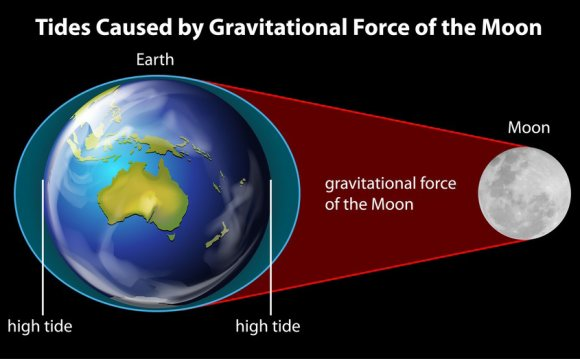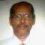# Gravitational pull between two objects

September 20, 2023Newton's Theory of Gravity states that every object in the universe pulls on every other object. Every object feels this force, so it is a universal force. The force is always attractive; it is always a pull, never a push. Picture the force of gravity as the tension in an imaginary rope between two objects. What factors determine the strength of the pull between two objects?

The masses of the two objects are important factors. Remember that mass is a measure of the amount of material in an object. Thus, mass is a measure of the number of "pullers" in that object. It makes sense that the more "pullers" there are, the stronger the pull. If we double the mass of one of the objects, we double the strength of the pull. If we double the masses of both objects, we quadruple the pull.

The other factor is not quite as easy to understand. The farther apart two objects become, the weaker the pull between the two becomes. Further, the relationship between distance and gravitational pull is not a simple one, but rather what we call an inverse square relationship. If, for example, we triple the distance between two objects, the gravitational force between them drops, not by three times, but by three times three times, or nine times.

Newton summarized the relationship between the strength of gravitation pull and these three factors in this equation:

Fg = G M1 M2 / (D12)2

where M1 and M2 are the masses of the objects, and D12is the distance between the centers of the two objects. G is a number called Newton's Universal Constant of Gravitation, which varies with the system of units we are using.

If we want to calculate absolute values of the gravitational force, in units of dynes or pounds or Newtons, we need to know the value of G. But that's not how Newton himself did it. We can get around knowing the value of G by comparing gravitational forces.

Here's how it's done. Imagine that we have four objects, numbered 1 through 4. How does the gravitational force between 1 and 2 compare to that between 3 and 4?

We must write out two different gravitational equations. First, one for the force between 1 and 2:

Fg12 = G M1 M2 / (D12)2

And now an equation for the force between 3 and 4:

Fg34 = G M3 M4 / (D34)2

Notice the use of subscripts to tell one equation apart from the other. Now, to compare the two forces, we divide one equation by the other:

Fg12 G M1 M2 / (D12)2
- = -
Fg34 G M3 M4 / (D34)2

Notice how, since G is in both the numerator and the denominator, it cancels out in this situation, and we don't have to worry about it. We can rearrange some of the factors that remain like so:

Fg12 / Fg34 = M1 M2 (D34)2/ M3 M4 (D12)2

Often, we will get a result that this ratio will equal some number. This indicates how the gravitational force between 1 and 2 compares to that between 3 and 4. For example, if we get

Fg12 / Fg34 = 10

Then we can say "The gravitational force between object 1 and object 2 is 10 times greater than the force between object 3 and object 4."

In many problems, we will make comparisons such that object 3 is the same as object 1, making calculations even simpler. We may also have a "before and after" situation, where object 4 is object 2 after some change, and object 3 is object 1 after some change. Examples of both are given below.

Source: www.austincc.edu
##### INTERESTING VIDEOGRAVITATIONAL PULL OF MY HEAD - PART 2Freely Falling Objects and Acceleration Due to Gravity##### INTERESTING FACTS##### What two factors determine the gravitational pull between two object

Mass and the distance between the two masses